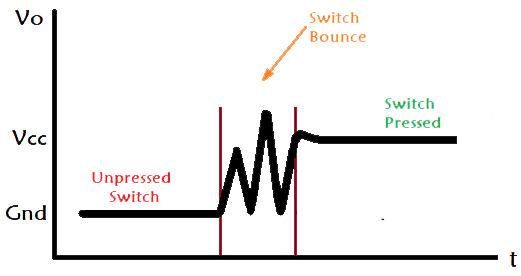# What causes tension in a rope moving in a vertical circle?

Rolacycle
Okay,i understand that force is smoke of acceleration but I intuitively can't understand what has caused this tension in rope like if their is a person who is whirling it I understand that person is providing the force similarly if car is turning over a curved road person who is driving it will rotate tyres to generate friction as to get centripetal accelaration.
It has to be secure on both ends otherwise load stay on ground. If there isn’t tension across pulley then there’s no point of the pulley. Tension happens when the force is applied to both ends.

Gold Member
I intuitively can't understand
Those four words sum up the OP's problem. No one can rely on intuition to 'understand' anything in this life. Politicians and snake oil salesmen work on that, every day. He has to leave that behind and do some learning - or thinking through what he's been told on this thread. That's better 'tuition' than 'in-tuition'.

•jbriggs444, Frigus and Rolacycle
Homework Helper
To me, constraint forces did not make a lot of intuitive sense until I put an intuitive mental model under them.

By themselves, constraint forces are not about causation. They are about correlation. If you have such and such an acceleration, you must have this much force. If you have such and such a force, you must have this much acceleration. Shut up and calculate.

The mental model that I personally put under a constraint force (e.g an object on the end of a rope) is that it is all about a stable equilibrium. Instead of an ideal rope with infinite stiffness, give the rope just a tiny bit of springiness. Now, if the object on the rope strays a little bit beyond the rope limit, the tension force gets higher. The result is that the object accelerates inward. It moves back to the rope limit. Similarly, if the object on the rope strays a little bit inside the rope limit, the tension force gets lower. Left to its own device, the object coasts back out to the rope limit.

By itself, this just means that there is an equilibrium point. But not necessarily a stable one. From experience, we know that things do not bounce back as fast as they left. There is always a little friction. Or hysteresis. Or whatever you want to call it. The equilibrium is stable. For a hemp rope (as opposed to a rubber band), the equilibrium will be approached quite rapidly.

This intuitive model does not need to be very quantitative. Or even very correct. It just has to be good enough to keep the intuition happy while one shuts up and calculates.

You might find that the concept of Operational Amplifiers is relevant. It's the same kind of mechanism. You have an underlying stable negative feedback loop that does not have to be very precise in order to yield a result which precisely follows a mathematical model.

•sophiecentaur and Frigus
Frigus
By themselves, constraint forces are not about causation. They are about correlation. If you have such and such an acceleration, you must have this much force. If you have such and such a force, you must have this much acceleration. Shut up and calculate.
I personally think that this is the key idea of Newtonian mechanics as there can be gazillions of situations in which some acceleration is there so if we keep finding how that mechanism happens for which we are solving then it can be quite painful and also will be out of context(I don't mean course here). For me acceleration is like smoke so if there is smoke then there should be fire somewhere which is force and we can find where the fire is just like what is the magnitude of force rather than try to understand about chemistry of fire.
This has already been stated in the thread. Have you just ignored it? Are you aware of the difference b between velocity and speed? (also stated earlier)
I was not talking about some finite distance but the distance it covers during the moments when rope is unstreched and streched.so it is obvious that ball's velocity will be change if their is some force which will be their when rope will be stretched.

Homework Helper
I was not talking about some finite distance but the distance it covers during the moments when rope is unstreched and streched.so it is obvious that ball's velocity will be change if their is some force which will be their when rope will be stretched.
Except for the first few moments while the system equilibriates, there is no dichotomy. The rope is not over-stretched or under-stretched. It is stretched just right.

We do not usually care about the initial bouncing about and do not have enough information to calculate it anyway. So we settle for figuring out the equilibrium force after things have settled down.

Once again, switching to the electronics arena for an analogy. Switch bounce:•sophiecentaur
•